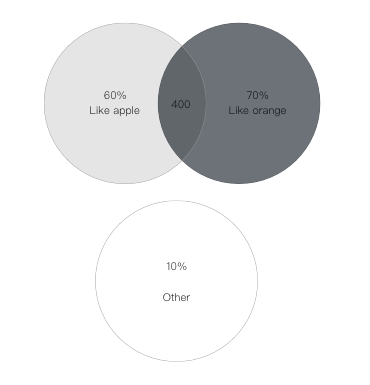¿Todavía tienes preguntas de matemáticas?

Pregunte a nuestros tutores expertos
Probability
Question

In a survey of a group of people, it was found that $$60\%$$ of the people liked apple, $$70\%$$ liked orange and $$400$$ people liked both of them. If $$10\%$$ people liked non of them, then

(i) Represent the above information in a Venn-diagram

(ii) Find the total number of people in the survey

(iii) Find the number of people who like apple only.(ii) $$1000$$
(iii) $$200$$